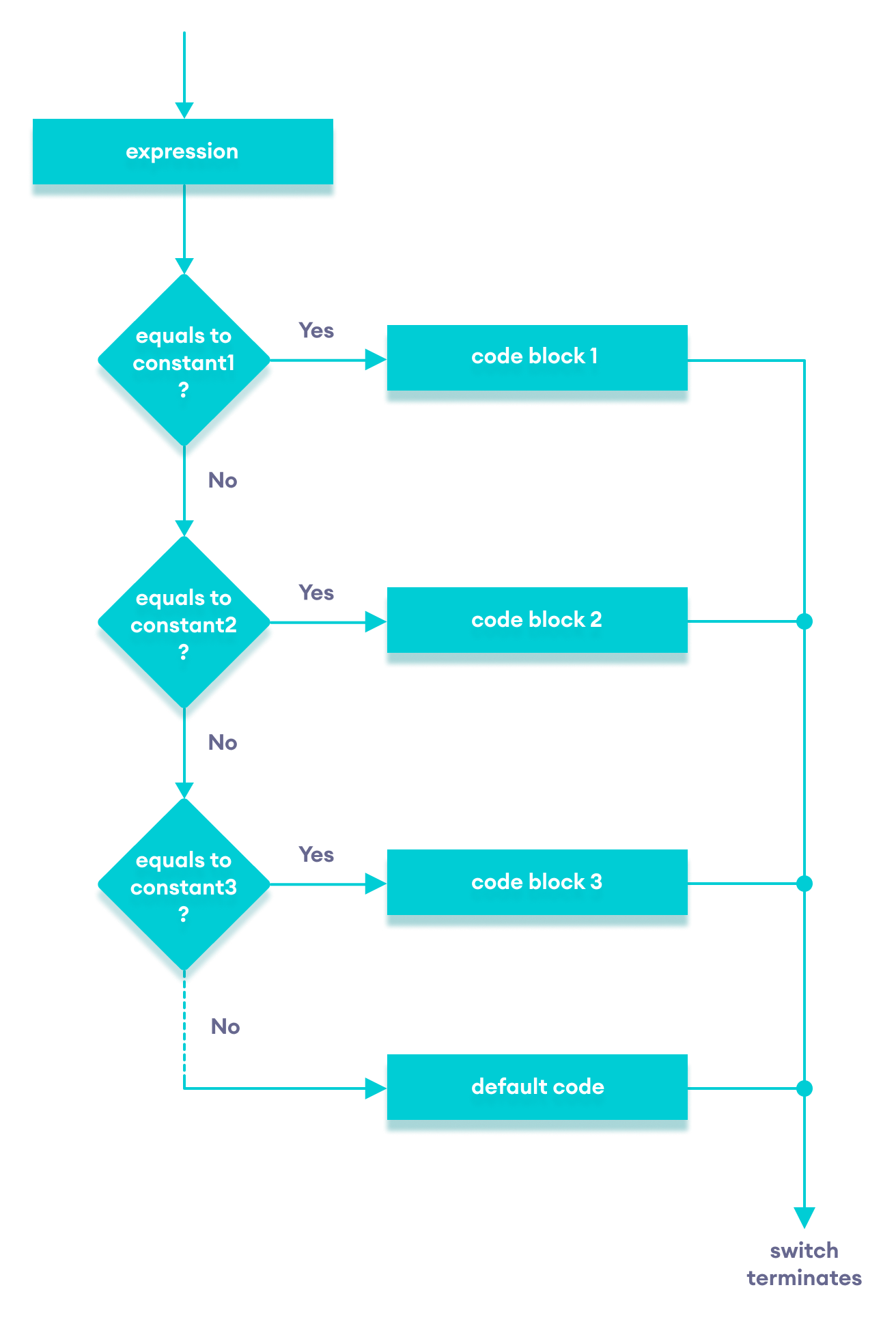# C++ switch..case Statement

In this tutorial, we will learn about switch statement and its working in C++ programming with the help of some examples.

The `switch` statement allows us to execute a block of code among many alternatives.

The syntax of the `switch` statement in C++ is:

``````switch (expression)  {
case constant1:
// code to be executed if
// expression is equal to constant1;
break;

case constant2:
// code to be executed if
// expression is equal to constant2;
break;
.
.
.
default:
// code to be executed if
// expression doesn't match any constant
}``````

How does the switch statement work?

The `expression` is evaluated once and compared with the values of each `case` label.

• If there is a match, the corresponding code after the matching label is executed. For example, if the value of the variable is equal to `constant2`, the code after `case constant2:` is executed until the break statement is encountered.
• If there is no match, the code after `default:` is executed.

Note: We can do the same thing with the `if...else..if` ladder. However, the syntax of the `switch` statement is cleaner and much easier to read and write.

## Flowchart of switch StatementFlowchart of C++ switch...case statement

### Example: Create a Calculator using the switch Statement

``````// Program to build a simple calculator using switch Statement
#include <iostream>
using namespace std;

int main() {
char oper;
float num1, num2;
cout << "Enter an operator (+, -, *, /): ";
cin >> oper;
cout << "Enter two numbers: " << endl;
cin >> num1 >> num2;

switch (oper) {
case '+':
cout << num1 << " + " << num2 << " = " << num1 + num2;
break;
case '-':
cout << num1 << " - " << num2 << " = " << num1 - num2;
break;
case '*':
cout << num1 << " * " << num2 << " = " << num1 * num2;
break;
case '/':
cout << num1 << " / " << num2 << " = " << num1 / num2;
break;
default:
// operator is doesn't match any case constant (+, -, *, /)
cout << "Error! The operator is not correct";
break;
}

return 0;
}``````

Output 1

```Enter an operator (+, -, *, /): +
Enter two numbers:
2.3
4.5
2.3 + 4.5 = 6.8```

Output 2

```Enter an operator (+, -, *, /): -
Enter two numbers:
2.3
4.5
2.3 - 4.5 = -2.2```

Output 3

```Enter an operator (+, -, *, /): *
Enter two numbers:
2.3
4.5
2.3 * 4.5 = 10.35```

Output 4

```Enter an operator (+, -, *, /): /
Enter two numbers:
2.3
4.5
2.3 / 4.5 = 0.511111```

Output 5

```Enter an operator (+, -, *, /): ?
Enter two numbers:
2.3
4.5
Error! The operator is not correct.```

In the above program, we are using the `switch...case` statement to perform addition, subtraction, multiplication, and division.

How This Program Works

1. We first prompt the user to enter the desired operator. This input is then stored in the `char` variable named oper.
2. We then prompt the user to enter two numbers, which are stored in the float variables num1 and num2.
3. The `switch` statement is then used to check the operator entered by the user:
• If the user enters `+`, addition is performed on the numbers.
• If the user enters `-`, subtraction is performed on the numbers.
• If the user enters `*`, multiplication is performed on the numbers.
• If the user enters `/`, division is performed on the numbers.
• If the user enters any other character, the default code is printed.

Notice that the `break` statement is used inside each `case` block. This terminates the `switch` statement.

If the `break` statement is not used, all cases after the correct `case` are executed.